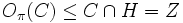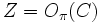# Pi-separable and pi'-core-free implies pi-core is self-centralizing

This article gives the statement, and possibly proof, of a particular subgroup of kind of subgroup in a group being self-centralizing. In other words, the centralizer of the subgroup in the group is contained in the subgroup
View other similar statements

## Statement

Suppose$\pi$ is a set of primes and$G$ is a finite group that is separable for the prime set$\pi$. Further, suppose the$\pi'$-core of$G$, namely$O_{\pi'}(G)$, is trivial. Then, the$\pi$-core of$G$, namely$O_\pi(G)$, is a self-centralizing subgroup of$G$:$\! C_G(O_\pi(G)) \le O_\pi(G)$.

## Facts used

1. Pi-separability is subgroup-closed
2. Characteristicity is centralizer-closed
3. Normality satisfies transfer condition
4. Characteristicity is transitive + Characteristic implies normal
5. Normal Hall implies permutably complemented: Note that this only uses the case where the normal Hall subgroup is abelian, which does not require the odd-order theorem.
6. Normality satisfies intermediate subgroup condition
7. Cocentral implies normal
8. Equivalence of definitions of normal Hall subgroup: A normal Hall subgroup is the same thing as a characteristic Hall subgroup.

## Proof

This proof uses a tabular format for presentation. Provide feedback on tabular proof formats in a survey (opens in new window/tab) | Learn more about tabular proof formats|View all pages on facts with proofs in tabular format

Given: A prime set$\pi$, a$\pi$-separable group$G$ such that$O_{\pi'}(G)$ is trivial; in other words,$G$ has no nontrivial normal$\pi'$-subgroup.

To prove:$C_G(O_{\pi}(G)) \le O_{\pi}(G)$.

Proof: Let$H = O_\pi(G)$ and$C = C_G(H)$. Let$Z = Z(H)$. By definition$Z = C \cap H$.

Step no. Assertion/construction Facts used Given data used Previous steps used Explanation
1$Z$ is normal in$C$ Fact (3) [SHOW MORE]
2$Z \le O_\pi(C)$ Step (2) [SHOW MORE]
3$O_\pi(C)$ is a normal$\pi$-subgroup of$G$ Facts (2), (4) [SHOW MORE]
4$O_\pi(C) \le C \cap H = Z$ [SHOW MORE]
5$Z = O_\pi(C)$: Steps (2), (4) Step-combination direct.
6 If$C$ is strictly bigger than$Z$, then$K = O_{\pi,\pi'}(C)$ is strictly bigger than$Z$ Fact (1)$G$ is$\pi$-separable. Step (5) [SHOW MORE]
7 If$C$ is strictly bigger than$Z$, there exists a nontrivial complement, say$M$, to$Z$ in$K$ Facts (5), (6) Steps (1), (6) [SHOW MORE]
8 If$C$ is strictly bigger than$Z$,$M$ is nontrivial normal Hall in$K$ Fact (7) Steps (6), (7) [SHOW MORE]
9 If$C$ is strictly bigger than$Z$,$M$ is a nontrivial normal$\pi'$-subgroup of$G$, i.e.,$M \le O_{\pi'}(G)$ Facts (2), (4), (8) Steps (6), (7), (8) [SHOW MORE]
10 If$C$ is strictly bigger than$Z$, we obtain the required contradiction to the assumption that$O_{\pi'}(G)$ is trivial. Thus,$C = Z$ and in particular we get$C \le H$, as desired.$G$ is$\pi'$-core-free. Steps (1), (9) Step (9) yields a nontrivial normal$\pi'$-subgroup of$G$, so$O_{\pi'}(G)$ is trivial.